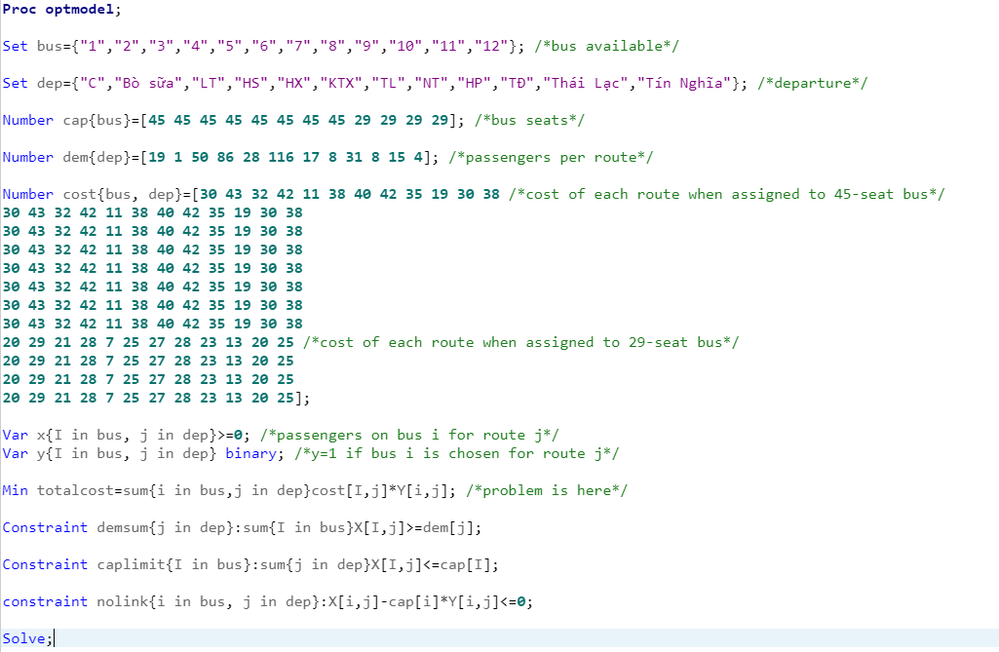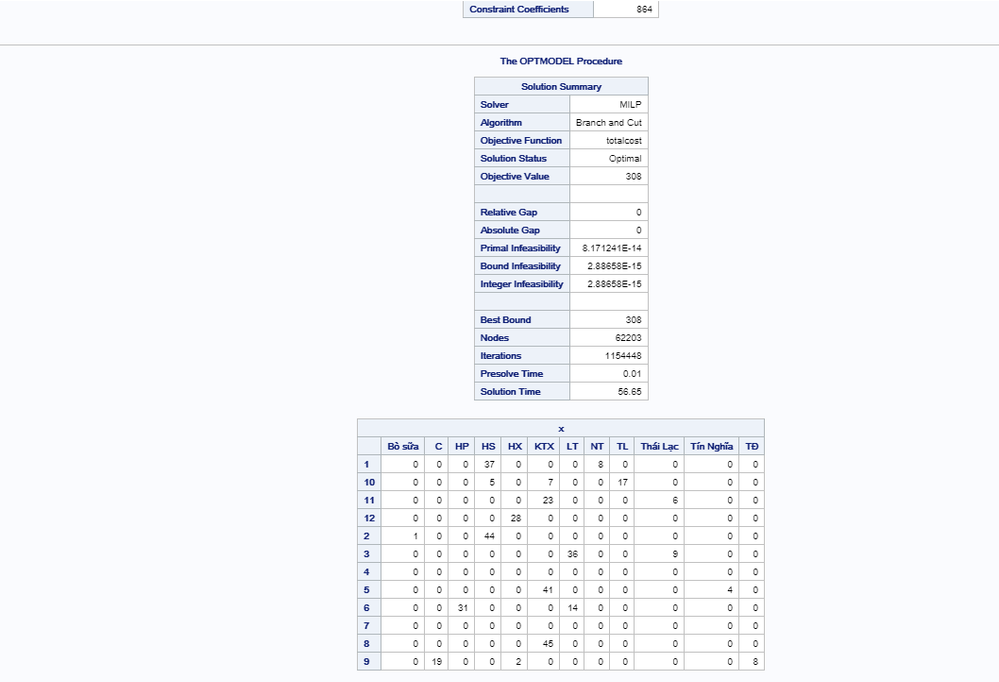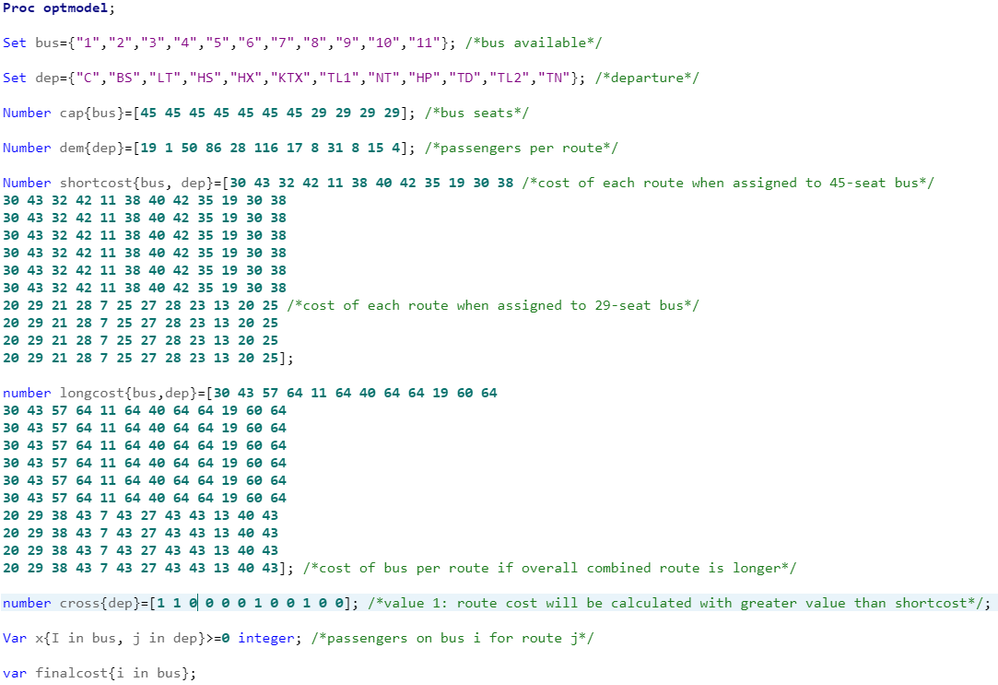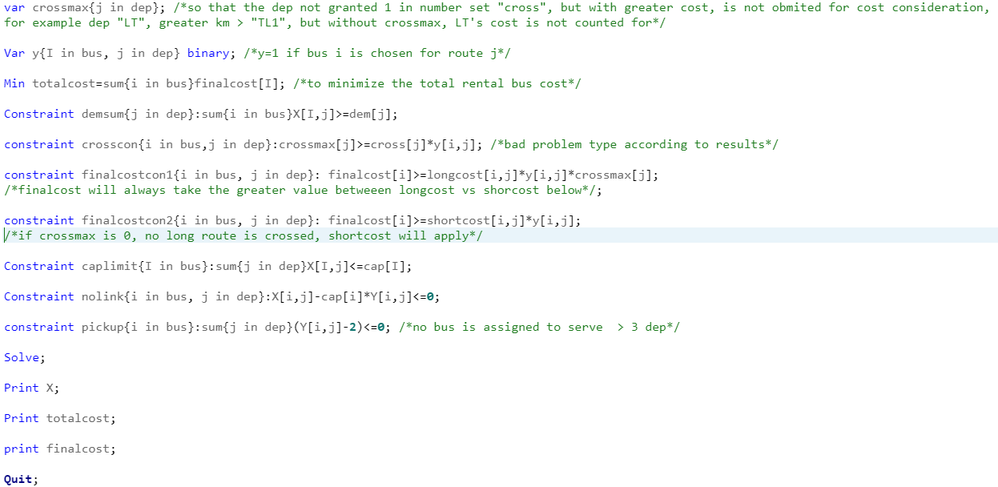## How do I determining max value in proc optmodel

Hi guys,

I am struggling with optmodel for bus arrangement optimization, the problem is to assign routes with different traveling cost to bus at lowest totalcost (1 bus can travel >1 route), and when different routes taken by a bus, the highest cost (among routes) will be the ultimate traveling cost for that bus.

My program looks like this up to now: (SAS studio for students)In Min (totalcost) formular, the totalcost is calculated with all cost of all routes taken by bus, which creates an overlap.

Please help me know if there is any way I can determine the max cost among routes for each bus.

Thank you very much indeed for your concern.

1 ACCEPTED SOLUTION

Accepted Solutions

## Re: How do I determining max value in proc optmodel

You have TL twice in the dep set, so rename the second one to, say, TL2 to avoid errors.

Your current objective is equivalent to the following:

``````var buscost{bus};
con buscostcon{i in bus}: buscost[i] = sum{j in dep} cost[i,j]*Y[i,j];
Min totalcost = sum{i in bus} buscost[i];
``````

It sounds like you want to change the buscost formula as follows:

``````con buscostcon{i in bus}: buscost[i] = max{j in dep} cost[i,j]*Y[i,j];
``````

The new constraint is nonlinear.  You can use SOLVE WITH LSO to get a good feasible solution.

But you can instead get an optimal solution by linearizing the constraint as follows:

``con buscostcon{i in bus, j in dep}: buscost[i] >= cost[i,j]*Y[i,j];``

This linear constraint guarantees that buscost[i] >= max{j in dep} cost[i,j]*Y[i,j].  Because the objective is minimization, this inequality will naturally be satisfied with equality at optimality.

8 REPLIES 8

## Re: How do I determining max value in proc optmodel

Please post your code as text if you want anyone to run and be able to test it. Or work with it.

## Re: How do I determining max value in proc optmodel

Thank you for reminding me, the code is posted below

## Re: How do I determining max value in proc optmodel

Here is my code, thank you:

Proc optmodel;

Set bus={"1","2","3","4","5","6","7","8","9","10","11","12"}; /*bus available*/

Set dep={"C","BS","LT","HS","HX","KTX","TL","NT","HP","TĐ","TL","TN"}; /*departure*/

Number cap{bus}=[45 45 45 45 45 45 45 45 29 29 29 29]; /*bus seats*/

Number dem{dep}=[19 1 50 86 28 116 17 8 31 8 15 4]; /*passengers per route*/

Number cost{bus, dep}=[30 43 32 42 11 38 40 42 35 19 30 38 /*cost of each route when assigned to 45-seat bus*/
30 43 32 42 11 38 40 42 35 19 30 38
30 43 32 42 11 38 40 42 35 19 30 38
30 43 32 42 11 38 40 42 35 19 30 38
30 43 32 42 11 38 40 42 35 19 30 38
30 43 32 42 11 38 40 42 35 19 30 38
30 43 32 42 11 38 40 42 35 19 30 38
30 43 32 42 11 38 40 42 35 19 30 38
20 29 21 28 7 25 27 28 23 13 20 25 /*cost of each route when assigned to 29-seat bus*/
20 29 21 28 7 25 27 28 23 13 20 25
20 29 21 28 7 25 27 28 23 13 20 25
20 29 21 28 7 25 27 28 23 13 20 25];

Var x{I in bus, j in dep}>=0; /*passengers on bus i for route j*/
Var y{I in bus, j in dep} binary; /*y=1 if bus i is chosen for route j*/

Min totalcost=sum{i in bus,j in dep}cost[I,j]*Y[i,j]; /*problem is here*/

Constraint demsum{j in dep}:sum{I in bus}X[I,j]>=dem[j];

Constraint caplimit{I in bus}:sum{j in dep}X[I,j]<=cap[I];

constraint nolink{i in bus, j in dep}:X[i,j]-cap[i]*Y[i,j]<=0;

Solve;

Print X;

Print Y;

## Re: How do I determining max value in proc optmodel

You have TL twice in the dep set, so rename the second one to, say, TL2 to avoid errors.

Your current objective is equivalent to the following:

``````var buscost{bus};
con buscostcon{i in bus}: buscost[i] = sum{j in dep} cost[i,j]*Y[i,j];
Min totalcost = sum{i in bus} buscost[i];
``````

It sounds like you want to change the buscost formula as follows:

``````con buscostcon{i in bus}: buscost[i] = max{j in dep} cost[i,j]*Y[i,j];
``````

The new constraint is nonlinear.  You can use SOLVE WITH LSO to get a good feasible solution.

But you can instead get an optimal solution by linearizing the constraint as follows:

``con buscostcon{i in bus, j in dep}: buscost[i] >= cost[i,j]*Y[i,j];``

This linear constraint guarantees that buscost[i] >= max{j in dep} cost[i,j]*Y[i,j].  Because the objective is minimization, this inequality will naturally be satisfied with equality at optimality.

## Re: How do I determining max value in proc optmodel

Your formula just worked out right, Prof.

Thank you very much indeed.

Sincerely.## Re: How do I determining max value in proc optmodel

Dear Sir,

Thank you for your help last time. The formula you introduced to me to determine the max value of a string is great, and I have applied it to extend my previous problem. This time, it is to change the cost of route into other value, when the route is combined with others (forcing travel on different route with greater distance, rendering higher cost).

Everything is fine with the max formula except for the crossmax, which I added in the end to make sure no route with greater cost is obmited for maxlongcost calculation. According to the result, the problem is a bad type, and I think it can be due to the crossmax.

I have checked all the way but still cannot figure out what's wrong with it.

It would be great if you could again help me with this.

Thank you very much for your concern.## Re: How do I determining max value in proc optmodel

I don't quite understand the cost rules, but the "Bad Problem Type" is because you are combining integer variables and nonlinear constraints without using SOLVE WITH LSO.  The nonlinearity arises from the product of variables y and crossmax here:

``constraint finalcostcon1{i in bus, j in dep}: finalcost[i]>=longcost[i,j]*y[i,j]*crossmax[j];``

Because the crosscon constraint and the minimization objective together imply that crossmax[j] > 0 only when y[i,j] = 1, you can linearize as follows:

``constraint finalcostcon1{i in bus, j in dep}: finalcost[i]>=longcost[i,j]*crossmax[j];``

``constraint pickup{i in bus}:sum{j in dep} Y[i,j] <= 2;``

Or just remove the parentheses from your version.

## Re: How do I determining max value in proc optmodel

Dear Sir, it worked again.
Thank you very much.
Discussion stats
• 8 replies
• 1387 views
• 3 likes
• 3 in conversation